Function Repository Resource:

Compute the additive digital root, in any base, of an integer

Contributed by: Christopher Stover
 ResourceFunction["AdditiveDigitalRoot"][n,b] gives the additive digital root of the non-negative integer n when expressed in the base b. ResourceFunction["AdditiveDigitalRoot"][n] gives the base-10 additive digital root of n.

## Details

Starting with a non-negative integer n, add its base-b digits, then add the digits of the resulting number, etc., until the result has only one digit. The one-digit integer marking the stopping point of this process is called the additive digital root of n.
As an example in base-10, consider n=1191:
Adding the digits of the result yields 1+2=3. Because 3 is a single-digit number, the process stops.
Because the integer marking the termination of the process is 3, ResourceFunction["AdditiveDigitalRoot"] returns 3.
The additive digital root is sometimes called the digital root (sans "additive"), and is denoted drb(n). It is also sometimes referred to as the repeated digital sum of n.

## Examples

### Basic Examples (2)

Compute the additive digital root of 1191:

 In:=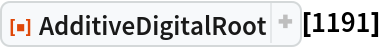Out=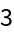Compute the additive digital root of 182, base-14 (note that the result has two digits base-10, but only a single base-14 digit):

 In:=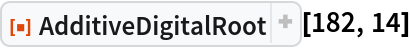Out=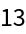### Scope (2)

 In:=Out=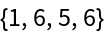In:=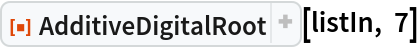Out=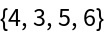Compute the base-10 additive digital roots of the first 100 integers (OEIS A010888):

 In:=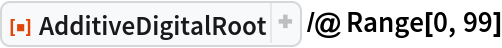Out=### Properties and Relations (5)

The additive digital root can be computed iteratively using NestWhile:

 In:=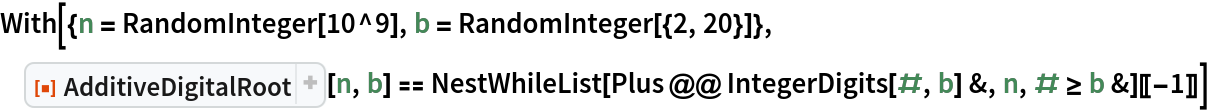Out=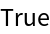The additive digital root can be computed using Floor:

 In:=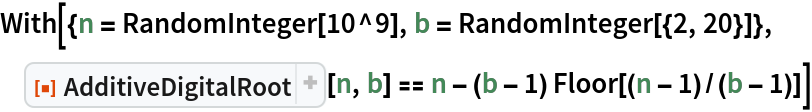Out=There is a well-known closed formula for the additive digital root in terms of Mod:

 In:=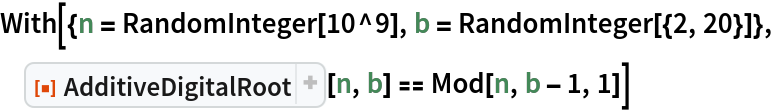Out=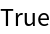The number of iterations required to reach the end of the digit addition process is called the additive persistence and is returned by the resource function AdditivePersistence:

 In:=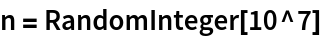Out=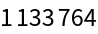In:=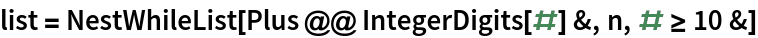Out=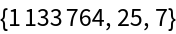In:=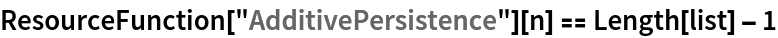Out=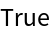The multiplicative analogue of the additive digital root is called the multiplicative digital root and is returned by the resource function MultiplicativeDigitalRoot:

 In:=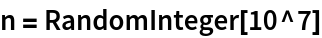Out=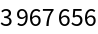In:=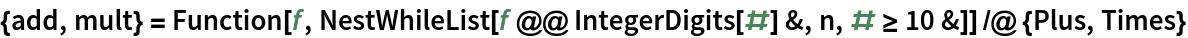Out=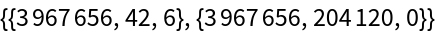In:=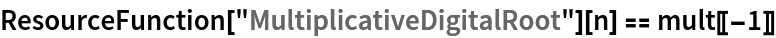Out=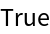### Possible Issues (1)

AdditiveDigitalRoot requires its input to be non-negative:

 In:=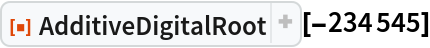Out=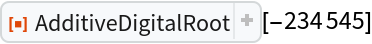## Version History

• 1.1.0 – 25 May 2023
• 1.0.0 – 09 August 2022

## Author Notes

Future versions of this will likely contain expansion to allow for integers in bases other than 10.